# JEE Main 2021 Analysis: Coordinate Geometry Made Easy, Important Questions And Solutions

###### ByRamraj Saini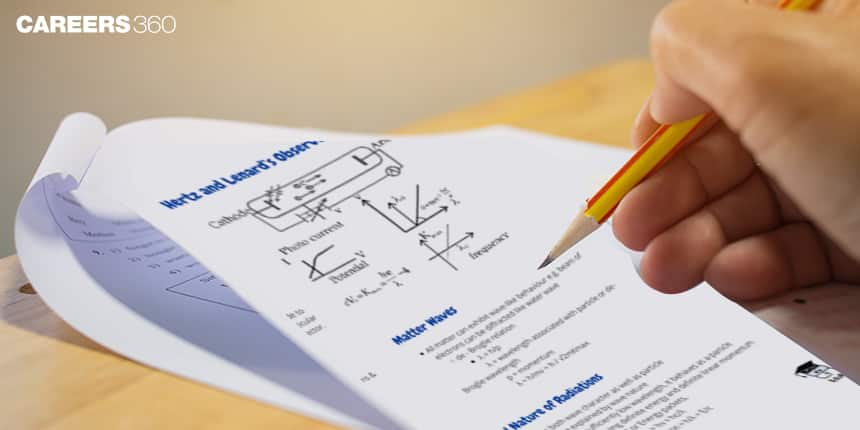###### Synopsis

Coordinate Geometry is an important part of JEE Main, the tough entrance exam for engineering admission to premier colleges. In JEE Main 2021 some 840 questions were from Mathematics and Coordinate Geometry accounted for 11.07 percent of them. Read through this article for a comprehensive analysis of Coordinate Geometry and know about the most important topics from where maximum questions were asked.###### Synopsis

Coordinate Geometry is an important part of JEE Main, the tough entrance exam for engineering admission to premier colleges. In JEE Main 2021 some 840 questions were from Mathematics and Coordinate Geometry accounted for 11.07 percent of them. Read through this article for a comprehensive analysis of Coordinate Geometry and know about the most important topics from where maximum questions were asked.

The National Testing Agency (NTA) has been conducting the Joint Entrance Examination (JEE) Main, Paper 1 for admission to undergraduate engineering programs (B.E/B.Tech.) at NITs, IIITs, and other premiere Institutes. Paper 1 is also an eligibility test for JEE Advanced, which is conducted for admission to IITs. Paper 2 is conducted for admission into B.Arch and B.Plan courses across the country.

The JEE Main syllabus consists of three subjects, that is, Physics, Chemistry, and Mathematics, and the Mathematics syllabus contains 16 chapters. JEE Main is a highly competitive exam and so aspirants should have a good command of each topic in every subject to score well in the exam. Mathematics has one-third weightage and therefore it becomes crucial to have a good hold on important topics in Mathematics syllabus. For a dedicated candidate, it is essential to have a winning plan that contains prioritizing important topics and analysis of the previous years’ papers to understand the breadth and depth of the topics.

This article analyzes Coordinate Geometry, one of the important Mathematics chapters of JEE Main 2021. It includes topics like Rectangular Cartesian Coordinate System, Distance Formula, Section Formula, Locus, Straight Lines, Circles, Conic Section, Parabola, Ellipse, Hyperbola, and some other topics as well. This article explains concepts with the help of previous years’ questions.

A total of 28 papers were held in both shifts in all the four sessions of JEE Main 2021. In these papers, 840 questions were from mathematics. Out of them 93 questions, that is, 11.07%, were from Coordinate Geometry. It shows how important this chapter is for JEE Main aspirants. The concepts, number of questions asked and weightage is given in the following table.

### Concepts And Number Of Questions

 Topic Number Of Questions Weightage (%) Circle 35 37.63 Parabola 17 18.27 Ellipse 12 12.90 Hyperbola 8 8.60 Line 42 45.16 Tangent 32 34.40 Normal 9 9.67 Locus 14 15.05 Area Of Triangle 11 11.82

It can be observed that out of 93 questions, concepts of a Line, Circle, Tangent are used in 42, 35 and 32 questions respectively. Concepts of the Circle contain standard and general forms of the equation of a circle, the equation of a circle when the endpoint of diameter is given, the intersection of circle and line, etc. Concepts of the line include an intersection of lines, the angle between two lines, condition for the intersection of three lines, coordinate of centroid, circumcenter, the orthocenter of a triangle, family of lines.

Solution of the following few questions from JEE Main 2021 show that command over multiple concepts is required to solve a problem. Practising every concept is essential as it can be difficult for students who are not aware or do not have a good hold on all the concepts to solve such questions.

Q.1 JEE Main 2021

The locus of a point, which moves such that the sum of squares of its distances from the points (0, 0), (1, 0), (0, 1), (1, 1) is 18 units, is a circle of diameter d. Then d2 is equal to

Solution

Let the point be P (h,k)

As per the question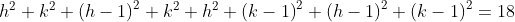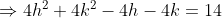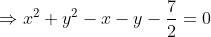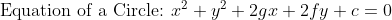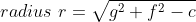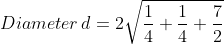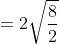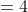Hence,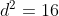Concepts Used

Distance formula, Concepts of locus, Equation of a circle.

Q.2 JEE Main 2021

Consider the parabola with vertex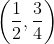and the directrix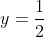. Let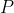be the point where the parabola meets the line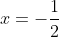. If the normal to the parabola at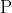intersects the parabola again at the point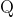, then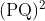is equal to.

Solution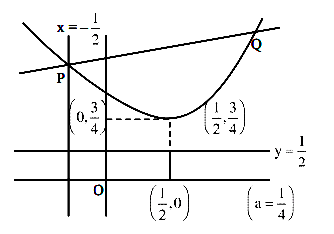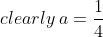Equation of parabola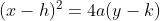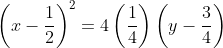For point P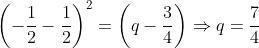For PQ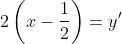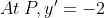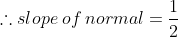Equation of PQ: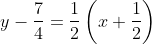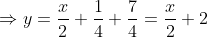For point Q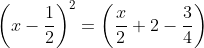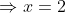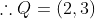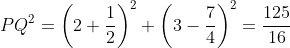Concepts Used

Intersection of line and parabola, Equation of a parabola, Concepts of the normal, Equation of a line in slope-intercept form, Distance formula.

Q.3 JEE Main 2021

The locus of mid-points of the line segments joining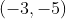and the points on the ellipse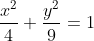are:

1.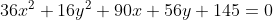2.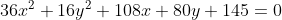3.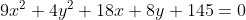4.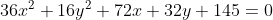Solution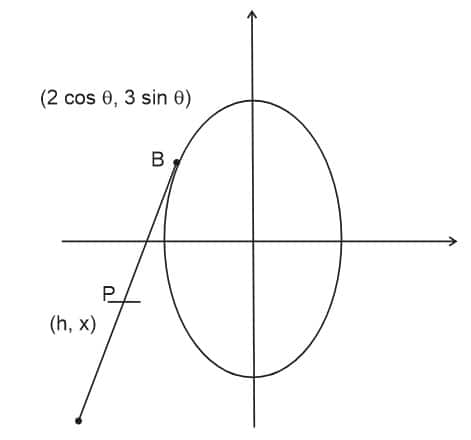Let us take any parametric point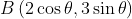on the ellipse

Let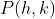be the midpoint of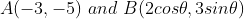So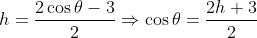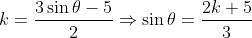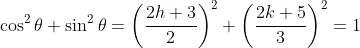Replace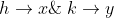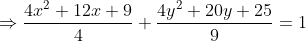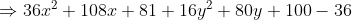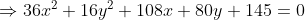Concepts Used

Point on the ellipse in parametric form, Concepts of locus.

Q.4 JEE Main 2021

The locus of the midpoints of the chords of the hyperbola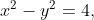which touch the parabola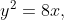is:

1.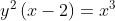2.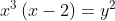3.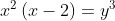4.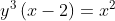Solution

Let the midpoint of chord be P(h,k)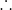equation of chord: T = S1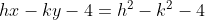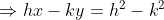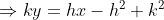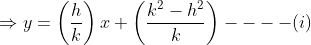Any tangent to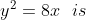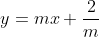Comparing it with (i)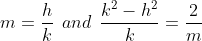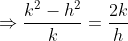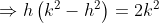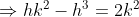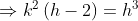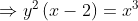Concepts Used

Equation of cord, Tangent on a parabola, Concepts of locus

Q.5 JEE Main 2021

The point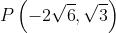lies on the hyperbola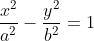having eccentricity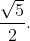If the tangent and normal at P to the hyperbola intersects its conjugate axis at the points Q and R respectively, then QR is equal to

1.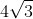2.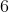3.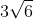4.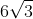Solution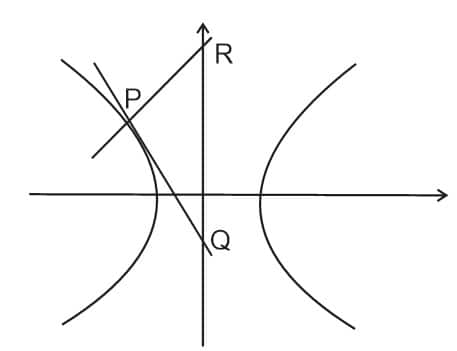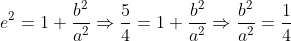and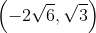lies on it, so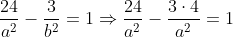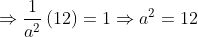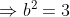Equation of the tangent at P:

T = 0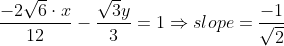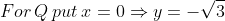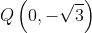Normal at p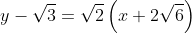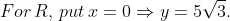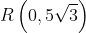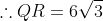Concepts Used

Equation of hyperbola, a point on hyperbola, tangent and normal on hyperbola, distance formula.

An analysis of the previous year's question shows that Coordinate Geometry questions can be solved very easily by having command over the basic concepts of each topic. Practising as many questions as possible, doing calculations fast without making any errors and practising NCERT book problems are also important. Lastly, solving problems from previous years’ JEE Main exam is highly beneficial to ace the JEE Main exam as it will let you know beforehand what sort of questions to expect and that can give you an edge over other competitors.

• Mathematics
• Mathematical objects
• Geometry
• Elementary mathematics
• Elementary geometry
• Euclidean geometry
• Analytic geometry
• Algebraic geometry
• Euclidean plane geometry
• Joint Entrance Examination

## Careers360 helping shape your Career for a better tomorrow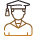#### 250M+

Students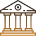#### 30,000+

Colleges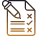#### 500+

Exams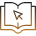E-Books